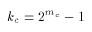# glBlendFunc

### NAME

glBlendFunc -- specify pixel arithmetic

### C SPECIFICATION

```void glBlendFunc(GLenum sfactor,
GLenum dfactor)
```

### PARAMETERS

sfactor
Specifies how the red, green, blue and alpha source blending factors are computed. Nine symbolic constants are accepted: GL_ZERO, GL_ONE, GL_DST_COLOR, GL_ONE_MINUS_DST_COLOR, GL_SRC_ALPHA, GL_ONE_MINUS_SRC_COLOR, GL_DST_ALPHA, GL_ONE_MINUS_DST_ALPHA, and GL_SRC_ALPHA_SATURATE.

dfactor
Specifies how the red, green, blue and alpha destination blending factors are computed. Eight symbolic constants are accepted: GL_ZERO, GL_ONE, GL_SCR_COLOR, GL_ONE_MINUS_SRC_COLOR, GL_SRC_ALPHA, GL_ONE_MINUS_SRC_COLOR, GL_DST_ALPHA, and GL_ONE_MINUS_DST_ALPHA.

### DESCRIPTION

In RGB mode, pixels can be drawn using a function that blends the incoming (source) RGBA values with the RGBA values that are already in the frame buffer (the destination values). By default, blending is disabled. Use glEnable and glDisable with argument GL_BLEND to enable and disable blending.

glBlendFunc defines the operation whenbelnding is enabled. sfactor specifies which of nine methods is used to scale the source color components. dfactor specifies which of eight methods is used to scale the destination color components. The eleven possible methods are described in the table below. Each method defines four scale factors, one for red, green, blue and alpha.

In the table and in subsequent equations, source and destination color components are referred to as (Rs, Gs, Bs, As) and (Rd, Gd, Bd, Ad). They are understood to have integer values between zero and (kR, kG, kB, kA), whereand (mR, mG, mB, mA) is the number of red, green, blue and alpha bitplanes.

Source and destination scale factors are referred to as (sR, sG, sB, sA) and (dR, dG, dB, dA). The scale factors described in the table, denoted (fR, fG, fB, fA) represent either source or destination factors. All scale factors have range [0, 1].In the table,

To determine the blended RGBA values of a pixel when drawing in RGB mode, the system uses the following equations:
Rd = min(kR, Rs*sR+Rd*dR)
Gd = min(kG, Gs*sG+Gd*dG)
Bd = min(kB, Bs*sB+Bd*dB)

Despite the apparent precision of the above equations, blending arithmetic is not exactly specified, because blending operates with imprecise integer color values. However, a blend factor that should be equal to one is guaranteed not to modify its multiplicand, and a blend factor equal to zero reduces its multiplicand to zero. Thus, for example, when sfactor is GL_SRC_ALPHA, dfactor is GL_ONE_MINUS_SRC_ALPHA, and As is equal to kA, the equations reduce to simple replacement:
Rd = Rs
Gd = Gs
Bd = Bs

### EXAMPLES

Transparency is best implemented using blend function (GL_SRC_ALPHA, GL_ONE_MINUS_SRC_ALPHA) with primitives sorted from farthest to nearest. Note that this transparency calculation does not require the presence of alpha bitplanes in the frame buffer.

Blend function (GL_SRC_ALPHA, GL_ONE_MINUS_SRC_ALPHA) is also useful for rendering antialiased points and lines in arbitrary order.

Polygon antialiasing is optimized using blend function (GL_SRC_ALPHA_SATURATE, GL_ONE) with polygons sorted from nearest to farthest. (See the glEnable, glDisable reference page and the GL_POLYGON_SMOOTH argument for information on polygon antialiasing.) Destination alpha bitplanes, which must be present for this blend function to operate correctly, store the accumulated coverage.

### NOTES

Incoming (source) alpha is correctly thought of as material opacity, ranging from 1.0 (kA), representing complete opacity, to 0.0 (0), representing completely transparency.

When more than one color buffer is enabled for drawing, blending is done separately for each enabled buffer, using for destination color the contents of that buffer. (See glDrawBuffer.)

Blending affects only RGB rendering. It is ignored by color index renderers.

### ERRORS

GL_INVALID_ENUM is generated if either sfactor or dfactor is not an accepted value.

GL_INVALID_OPERATION is generated if glBlendFunc is called between a call to glBegin and the corresponding call to glEnd.

### ASSOCIATED GETS

glGet with argument GL_BLEND_SRC
glGet with argument GL_BLEND_DST
glIsEnabled with argument GL_BLEND